Include Top

# Exponential Smoothing Forecast

## Simple Exponential Smoothing

Simple Exponential Smoothing forecasts are calculated using weighted averages, where the weights decrease exponentially as observations come from further in the past with the smallest weights associated with the oldest observations:Error, Trend, Seasonal (ETS) Models

Error, Trend, Seasonal (ETS) models expand on simple exponential smoothing to accommodate trend and seasonal components as well as additive or multiplicative errors. Simple Exponential Smoothing is an Error Model. Error, Trend model is Holt’s Linear, also known as double exponential smoothing. Error, Trend, Seasonal model is Holt-Winters, also known as triple exponential smoothing. Rob Hyndman has developed a complete taxonomy that describes all of the combinations of exponential smooth models in a consistent manner (see fpp2):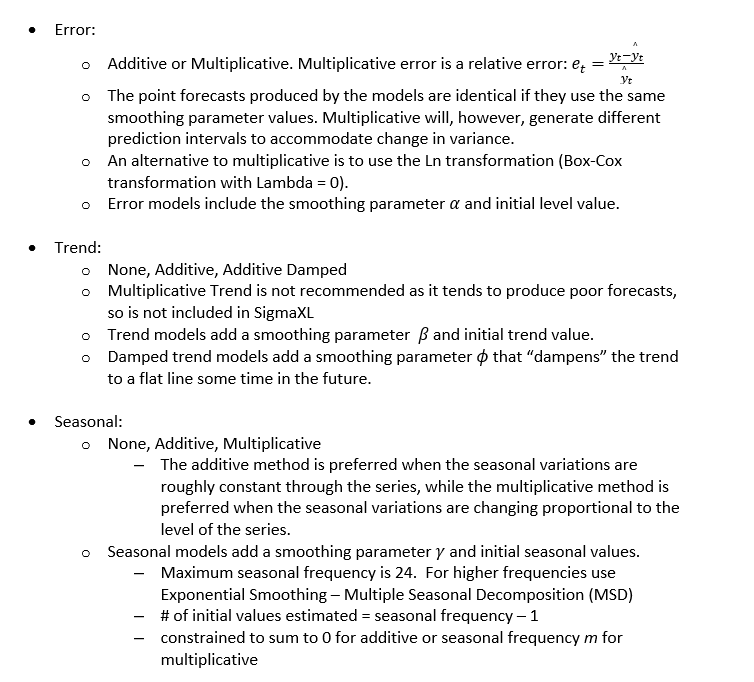For further details, see Appendix: Exponential Smoothing - ETS.

Summary of ETS Models in SigmaXL: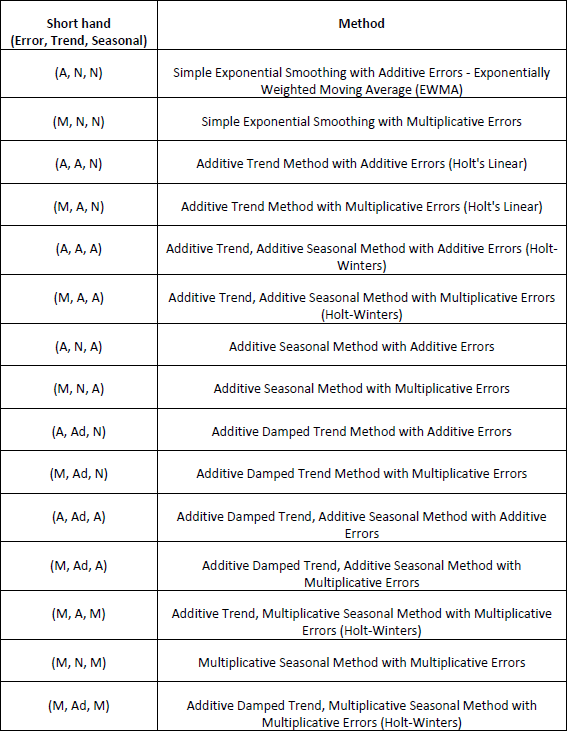Exponential Smoothing Parameter Estimation, Model Statistics and Information Criteria for Model Comparison

Model parameters are solved using nonlinear maximization of the Log-Likelihood function. Log-Likelihood is related to -Ln(Sum-of-Squares Error). Information Criteria AICc, AIC and BIC are calculated using -2*Log-Likelihood and incorporate a penalty for the number of terms in the model, so smaller is better. These are used in automatic model selection. AICc is the default Information Criterion, based on forecast error performance with competition data.

Missing Values

Exponential Smoothing handles missing values with seasonally adjusted linear interpolation. While there is robustness against some missing values, if the number of missing values is large then model estimation and forecast accuracy will be degraded. Upon selection of a time series with missing values, you will see a pop-up “Warning: Missing values detected. Seasonally adjusted linear interpolation will be used.”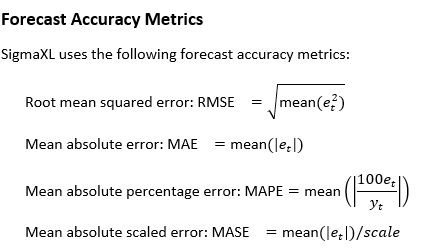where scale is the MAE of the in-sample naïve or seasonal naïve forecast (set all forecasts to be the value of the last observation/period). Note that scale for nonseasonal is identical to MR-bar of an Individuals Moving Range chart. A scaled error is less than one if it arises from a better forecast than the average naïve/seasonal naïve forecast. Conversely, it is greater than one if the forecast is worse than the average naïve forecast. MASE is recommended over the popular MAPE, because MAPE becomes infinite if any y_t=0.

In-Sample is also referred to as the “Train” data. The same metrics may be applied to Out-of-Sample (Withhold), also referred to as the “Test” data and may be One-Step-Ahead or Multi-Step-Ahead. This will be demonstrated later. Out-of-Sample (Withhold) data is not used in the model parameter estimation, so is a much better indicator of true forecast accuracy. In-sample accuracy metrics can be biased due to overfitting. Scale is always computed using the in-sample data.

## Demo of Simple Exponential Smoothing – Concentration

1. Open Demo of Simple Exponential Smoothing – Concentration.xlsx (Sheet 1 tab). This is the Series A data from Box and Jenkins, a set of 197 concentration values from a chemical process taken at two-hour intervals in Column B.

2.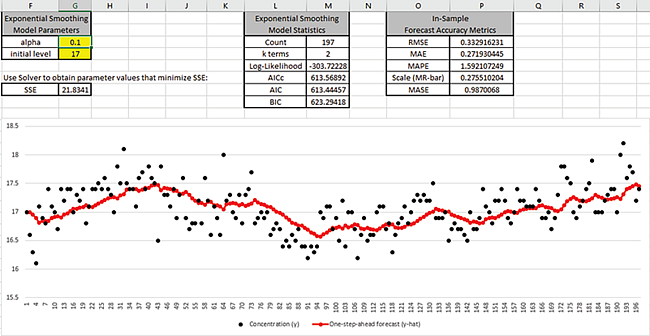Cell G3 is the alpha smoothing parameter value, cell G4 is the initial level. These may be manually entered or solved with Solver. The One-step-ahead forecast is calculated as follows: C2 is the initial level value; C3 =alpha*B2+(1-alpha)*C2; C4 =alpha*B3+(1-alpha)*C3, etc.

Cell G7 is the Residuals Sum-of-Squares error and is the value to be minimized, in order to produce the most accurate one-step-ahead forecast. The exponential smooth model statistics are also given for later comparison to the SigmaXL report.

The raw Concentration data are the black dots in the graph; the one-step-ahead forecast values are the red dots. Model Statistics and Forecast Accuracy Metrics will be discussed later.

3. Before we use Solver to determine the optimal alpha and initial level values, we will manually enter some values to see how the graph changes. Enter alpha = 0.01 as shown:

4.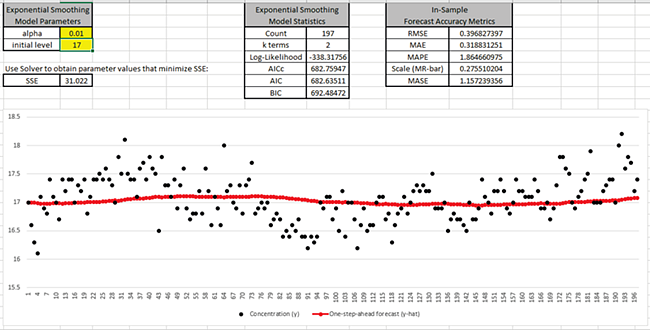This small value of alpha produces a smooth fit with SSE = 31.02 that is larger than what we started with (SSE = 21.83).

5. Now enter alpha = 0.99 as shown:

6.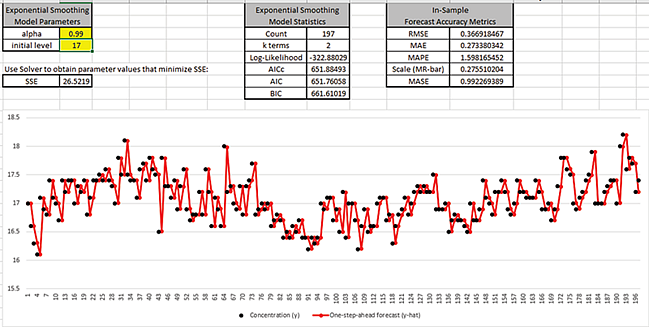This large value of alpha results approximately in a naïve forecast, with the one-step-ahead forecast value equal to the previous actual. SSE = 26.52 is larger than what we started with.

7. Enter alpha = 0.1 and initial level = 20 as shown:

8.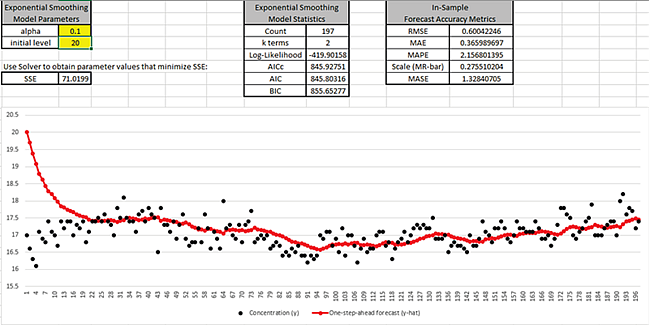This initial level is a poor estimate of the start value and results in SSE = 71.02. After about 30 observations, the influence of this poor start value is negligible. The duration for which the initial level has influence on the one-step-ahead forecast depends on alpha, a smaller alpha would be a longer duration; a larger alpha would be a shorter duration.

9. Now we will use Excel’s Solver to find the optimal values for alpha and initial level. Click Data > Solver. (If the Solver menu is not available, click File > Options > Add-Ins. Click Go… Manage Excel Add-ins, check Solver Add-in. Click OK. Click Data > Solver.)

10.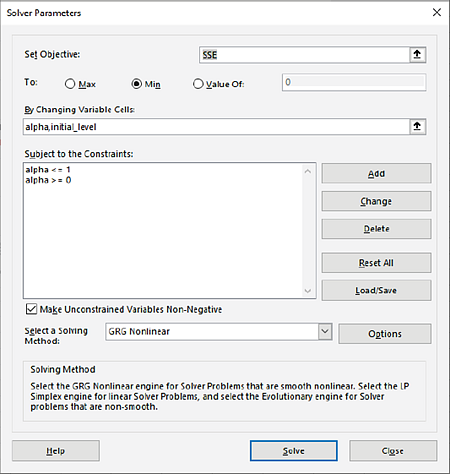The cell addresses, constraints and settings for Solver have been stored in the workbook, so no changes are necessary. Solver will minimize cell G7 (SSE), by varying cells G3 (alpha) and G4 (initial_level). The solving method is GRG Nonlinear; Evolutionary could also be used but it is slower.

11. Click Solve. The Solver Results dialog shows that a solution has been found.

12.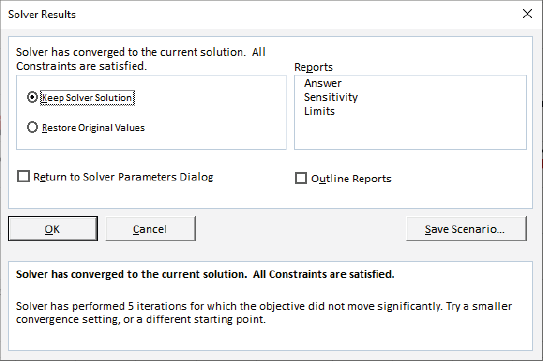13. Click OK. The SSE is now 19.74; alpha value = 0.295 and initial level = 16.732.

14.By minimizing the residual sum-of-squares error (SSE), we have our best estimate of the parameters to produce one-step-ahead forecast values.

15. We will now produce ACF/PACF plots for the Residuals. Select cells D1:D198 and click SigmaXL > Time Series Forecasting > Autocorrelation (ACF/PACF) Plots. Click Next, Select Residuals (e), Click OK.

16.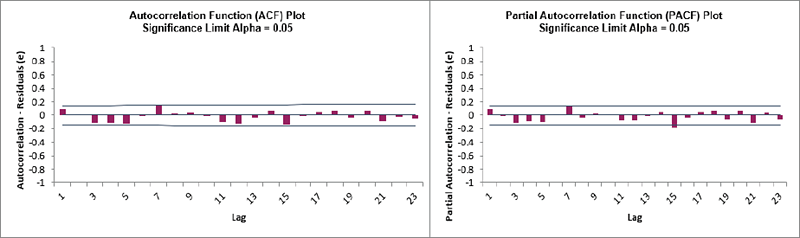We can see that all of the autocorrelation has been removed by the simple exponential smoothing model (with the exception of lag 15 in the PACF), so this is a good fit to the time series data.

17. By way of comparison, these are the ACF/PACF plots for the original Concentration data that we produced earlier.

18.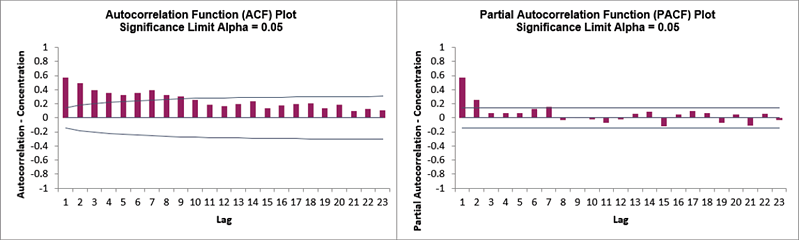19. We will now have a quick look at the Residuals using a Histogram. Select the Residuals data (D1:D198). Click SigmaXL > Graphical Tools > Histograms and Descriptive Statistics. Next, Select Residuals (e), Click OK.

20.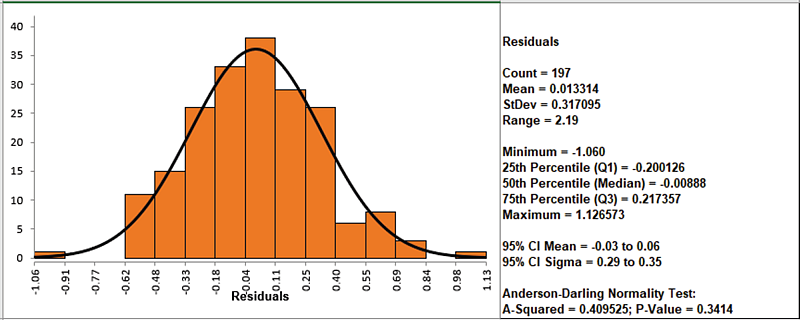The residuals are normally distributed with no obvious extreme outliers.

## Chemical Process Concentration - Series A

1. Open Chemical Process Concentration – Series A.xlsx (Sheet 1 tab). This is the Series A data from Box and Jenkins, a set of 197 concentration values from a chemical process taken at two-hour intervals. See the Run Chart, ACF/PACF Plots, Spectral Density Plot and Seasonal Trend Decomposition Plots for this data.

2. Click SigmaXL > Time Series Forecasting > Exponential Smoothing Forecast > Forecast. Ensure that the entire data table is selected. If not, check Use Entire Data Table. Click Next.

3. Select Concentration, click Numeric Data Variable (Y) >>. Check Display ACF/PACF/LB Plots and Display Residual Plots. Leave Specify Model Periods, Seasonal Frequency and Box-Cox Transformation unchecked. We will use the default No. of Forecast Periods = 24 and Prediction Interval = 95.0 %.

4.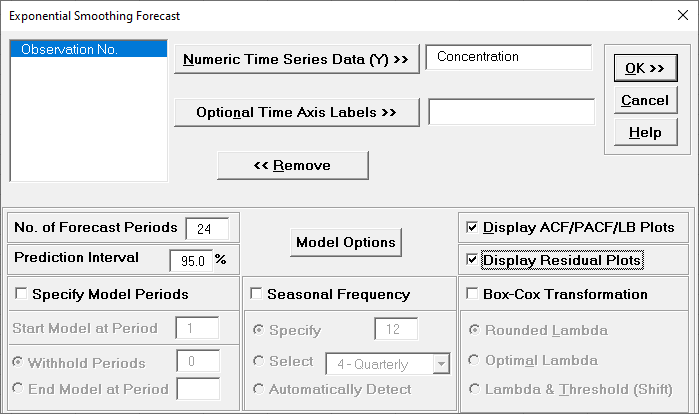Optional Time Axis Labels will be displayed on the forecast chart time axis. If used, dates for the forecast periods should also be included, otherwise the time axis will be blank for the forecast periods.

No. of Forecast Periods are the number of time series values to be predicted (forecast horizon). The most accurate forecast will be for the first predicted value (one-step-ahead).

Prediction Interval % is the confidence level for the individual predictions. For example, a 95% prediction interval contains a range of values which should include the actual future value with 95% probability. The interval will get larger the further out you predict.

Model Options opens another dialog which allows you to set automatic options or to specify a model.

Display ACF/PACF/LB option will produce ACF and PACF plots for the raw data as well as for the model residuals. The LB plot is a plot of Ljung-Box test P-Values for various lags and is used to determine if a group of autocorrelations are significant, (i.e., the autocorrelations do not come from a white noise series).

Display Residual Plots will produce a table of model residuals and the usual model residual plots: histogram, normal probability plot, residuals versus data order, and residuals vs forecast value. Note that if a Box-Cox transformation is applied, the residuals are transformed so will not be equal to forecast - actual.

Specify Model Periods are used to specify a start period, end period or withhold sample. The withheld data is not used in model estimation, so this is very useful for model validation and comparison. This will be used in a later example.

Seasonal Frequency and Box-Cox Transformation will be used in a later example.

5. Click Model Options.

6.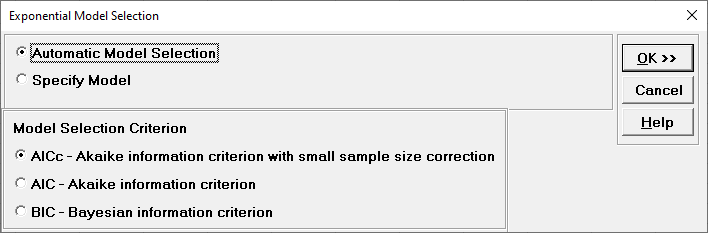Automatic Model Selection will be used later. It is the default selection.

Model Selection Criterion is the information criterion metric to be used in automatic model selection. AICc is the default selection.

• Clicking OK accepts the settings and returns you to the previous dialog. Clicking Cancel will cancel any changes and return you to the previous dialog.

7. Select Specify Model.

8.Specify Model allows you to manually specify the Error-Trend-Seasonal model. Seasonal options are greyed out because the Seasonal Frequency option in the main dialog is unchecked. A summary description of the model is also given. This will be included in the forecast report.

9. We will use the default Error: Additive and Trend: None, which is a simple exponential smoothing model, the same as was demonstrated previously. Click OK to return to the Exponential Smoothing Forecast dialog. Click OK. The exponential smoothing forecast chart is given:

10.This is very similar to the exponential smooth plot demonstrated above, showing the raw Concentration data (black) and one-step-ahead forecast values (red), but with the addition of a 24-period forecast and the 95% prediction interval.

11. You can zoom in to view the last 30 points on the Forecast Chart. Click SigmaXL Chart Tools > Show Last 30 Data Points.

12.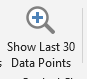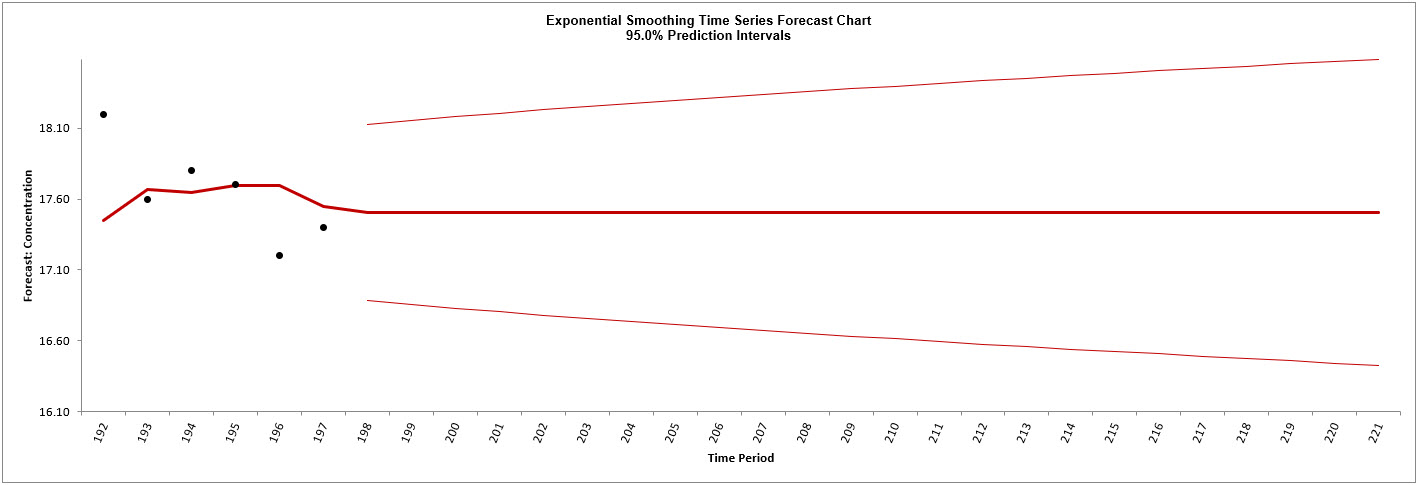13. To restore the chart, click SigmaXL Chart Tools > Show All Data Points.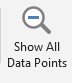14. You can also scroll through the data. Click SigmaXL Chart Tools > Enable Scrolling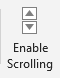You are prompted with a warning message that custom formatting on the chart will be cleared:You can avoid seeing this warning by checking Save this choice as default and do not show this form again.

15. Click OK. The scroll dialog appears allowing you to specify the Start Period and Window Width:

16.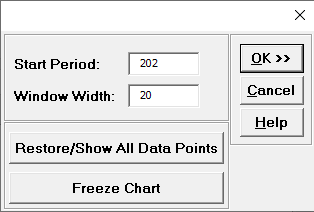At any point, you can click Restore/Show All Data Points or Freeze Chart. Freezing the chart will remove the scroll and unload the dialog. The scroll dialog will also unload if you change worksheets. To restore the dialog, click SigmaXL Chart Tools > Enable Scrolling.

17. Click OK. A scroll bar appears beneath the forecast chart. You can also change the Start Subgroup and Window Width and Update.

18.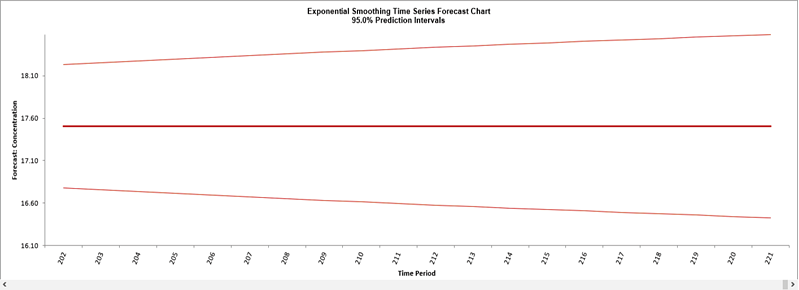You can scroll through by clicking to the right or left, with the specified window width of 20. Click left once to view the chart as shown.

19. Click Cancel to exit the scroll dialog.

20. Scroll down to view the Exponential Smoothing Model header:

21.The model Simple Exponential Smoothing with Additive Errors (A, N, N) – Exponentially Weighted Moving Average (EWMA) is the user specified model. This is the same model information that was displayed in the Model Selection dialog.

If we had checked Specify Model Periods in the main dialog, the start, end or withhold selection would be summarized here as well.

22. The Exponential Smoothing Model Information is given as:

23.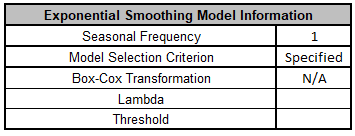This is a summary of model information with Seasonal Frequency = 1 (nonseasonal); Model Selection Criterion = “Specified” because the model was user specified; and Box-Cox Transformation = “N/A” because Box-Cox Transformation was unchecked.

24. The Parameter Estimates are:The parameter estimates closely match the values obtained earlier in the demonstration using Solver: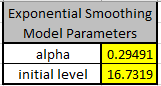The slight differences in parameter results are due to differences in optimization method.

25. The Exponential Smoothing Model Statistics are:Degrees of freedom (DF) = n – 2 (terms in the model). See Exponential Smoothing Parameter Estimation, Model Statistics and Information Criteria for Model Comparison.

The results match those given in the demonstration using Solver: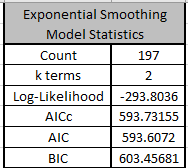The equations may be viewed by clicking on the Demo cells M5, M6, M7 or M8.

26. The In-Sample Forecast Accuracy metrics are: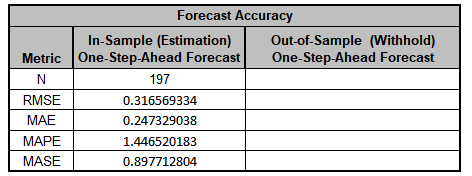MASE is less than one, so it is a better forecast than would be obtained from a naïve forecast (set all forecasts to be the value of the last observation). See Forecast Accuracy Metrics. The results closely match those given in the demonstration using Solver: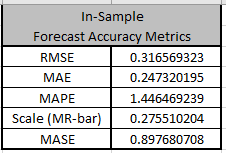The equations may be viewed by clicking on the Demo cells P3 to P7.

27. The Forecast Table is given as:These are the same forecast and prediction interval values displayed in the Forecast Chart but provided for further analysis or charting. If Withhold Periods are specified, the Withhold Data will be displayed as well.

28. Click on the Exp. Smooth. ACF PACF LB sheet to view the ACF/PACF/LB Plots:These match the plots that we obtained previously in the Demo. We can see that all of the autocorrelation has been removed by the exponential smoothing model (with the exception of lag 15 in the PACF), so this is a good fit to the time series data.The LB plot is a plot of Ljung-Box test P-Values for various lags and is used to determine if a group of autocorrelations are significant, (i.e., the autocorrelations do not come from a white noise series). For further details, see Ljung-Box Test.

The red P-Values are significant (alpha=.05) and the blue P-Values are not significant. It is desirable that all P-Values be blue. The ACF/PACF plots indicated that almost all of the correlation has been accounted for in the model, but the Ljung-Box plot shows that some significant autocorrelation still remains - so the model can potentially be improved. This does not mean that the model is a bad model, it can still be very useful for prediction purposes, but the prediction intervals may not provide accurate coverage.

29. Click on the Exp. Smoothing Residuals sheet to view the Residual Plots:These residual plots are the same as used in SigmaXL’s Multiple Regression. The histogram matches what we obtained in the Demo (the normal curve is not applied here). The residuals are approximately normally distributed, with a roughly straight line on the normal probability plot. There are no obvious extreme outliers or patterns in the charts. Later, we will apply a control chart to the residuals to formally test for significant outliers or assignable causes.

30. Now we will rerun Exponential Smoothing on the Concentration data but use Automatic Model Selection. Click Recall SigmaXL Dialog menu or press F3 to recall last dialog.31. Click Model Options. Select Automatic Model Selection. We will use the default Model Selection Criterion: AICc – Akaike information criterion with small sample size correction.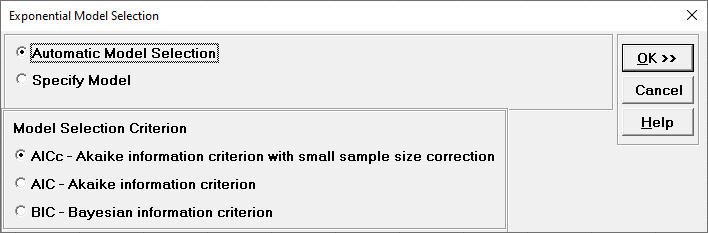32. Click OK to return to the Exponential Smoothing Forecast dialog. Click OK. The exponential smoothing forecast report is given.

33. Scroll down to view the Exponential Smoothing Model header: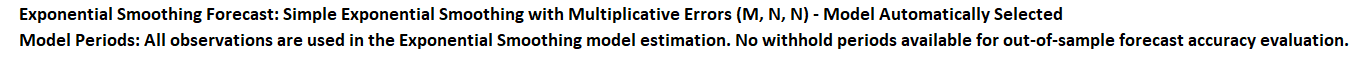The model Simple Exponential Smoothing with Multiplicative Errors (M, N, N) was selected as the best fit for the Concentration data based on the AICc criterion. The point forecasts produced by the Multiplicative and Additive models are identical if they use the same smoothing parameter values. Multiplicative will, however, generate different prediction intervals to accommodate change in variance.

34. The Exponential Smoothing Model Information is given as:This is a summary of model information with Seasonal Frequency = 1 (nonseasonal); Model Selection Criterion = “AICc” and Box-Cox Transformation = “N/A” because Box-Cox Transformation was unchecked.

35. The Parameter Estimates are:These are fairly close to the parameter estimates obtained above using the additive model.

36. The Exponential Smoothing Model Statistics are: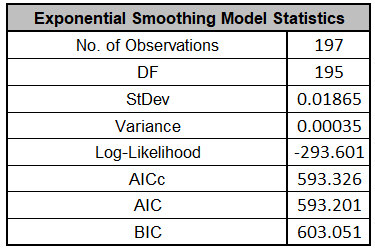The Log-Likelihood, AICc, AIC and BIC are close to the values obtained above using the additive model, but the Log-Likelihood is slightly higher giving a lower AICc, so multiplicative was selected as the best model. Note however that the StDev and Variance are very different. This difference is due to the multiplicative residuals being relative errors: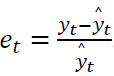37. The In-Sample Forecast Accuracy metrics, Forecast Table and ACF/PACF/LB Plots for multiplicative are very close to the additive model. The multiplicative residual plots look the same, but note the different scale due to the relative errors.38. Now we will rerun Exponential Smoothing on the Concentration data with Automatic Model Selection, but will use Specify Withhold Periods. Click Recall SigmaXL Dialog menu or press F3 to recall last dialog. Check Specify Model Periods. Set Withhold Periods = 24 (i.e., we will forecast 24 periods and compare against the withheld actual). We will use the default Withhold Forecast Type: One-Step-Ahead with Prediction Interval at: Start of Withhold.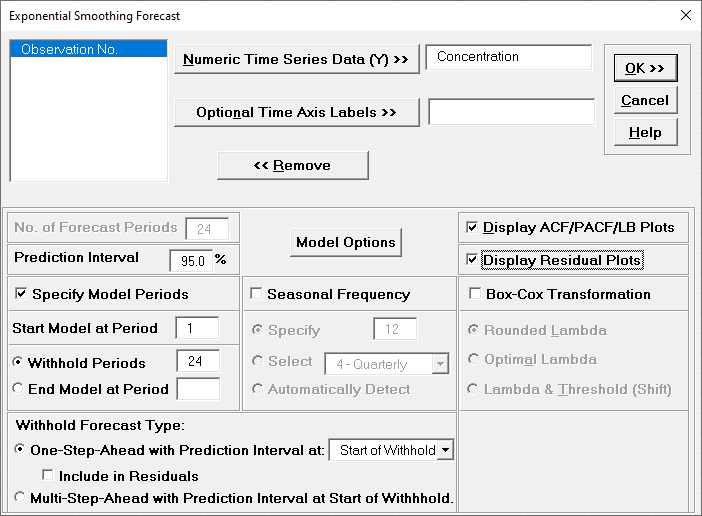Specify Model Periods option allows you to specify the start and end periods used in automatic model identification and parameter estimation. Typically, Start Model at Period is kept = 1 and Withhold Periods specifies the number of periods to be withheld for out-of-sample testing. End Model at Period specifies the end period, so the withhold sample size would be: total number of observations – end period.

Withhold Forecast Type: One-Step-Ahead will exclude the withhold sample from automatic model identification and parameter estimation, but uses the withhold data to update the predicted one-step ahead forecast. This is useful to assess forecast error when you only care about the short-term one-step ahead prediction.

Withhold Forecast Type: One-Step-Ahead with Prediction Interval at: Start of Withhold will display the prediction interval for the duration of the withhold sample. Note that the length of the prediction interval is determined by the number of withhold periods, so overrides the specified No. of Forecast Periods.

Withhold Forecast Type: One-Step-Ahead with Prediction Interval at: End of Withhold will display the prediction interval at the end of the withhold sample. The length of the prediction interval is determined by the specified No. of Forecast Periods.

Include in Residuals will treat the one-step-ahead forecast errors as residuals (even though they were not part of the model estimation process) and will be included in the ACF/PACF/LB Residual Plots along with the Residuals report and graphs. Typically, this is kept unchecked.

Withhold Forecast Type: Multi-Step-Ahead with Prediction Interval at Start of Withhold will exclude the withhold sample from automatic model identification and parameter estimation and does not use the withhold data to update the predicted one-step ahead forecast. This is useful to assess forecast error when you are interested in a long-term forecast window (horizon). The prediction interval will be displayed for the duration of the withhold sample. Note that the length of the prediction interval is determined by the number of withhold periods, so overrides the specified No. of Forecast Periods. These forecast errors are not included in ACF/PACF/LB Residual Plots or the Residuals report and graphs.

39. Click Model Options. Select Automatic Model Selection. We will use the default Model Selection Criterion: AICc – Akaike information criterion with small sample size correction.Tip: When using Recall SigmaXL Dialog, and if there are no changes to the Model Option settings, the previous settings will be used. It is not necessary to repeat this step.

40. Click OK to return to the Exponential Smoothing Forecast dialog. Click OK. The exponential smoothing forecast report is given: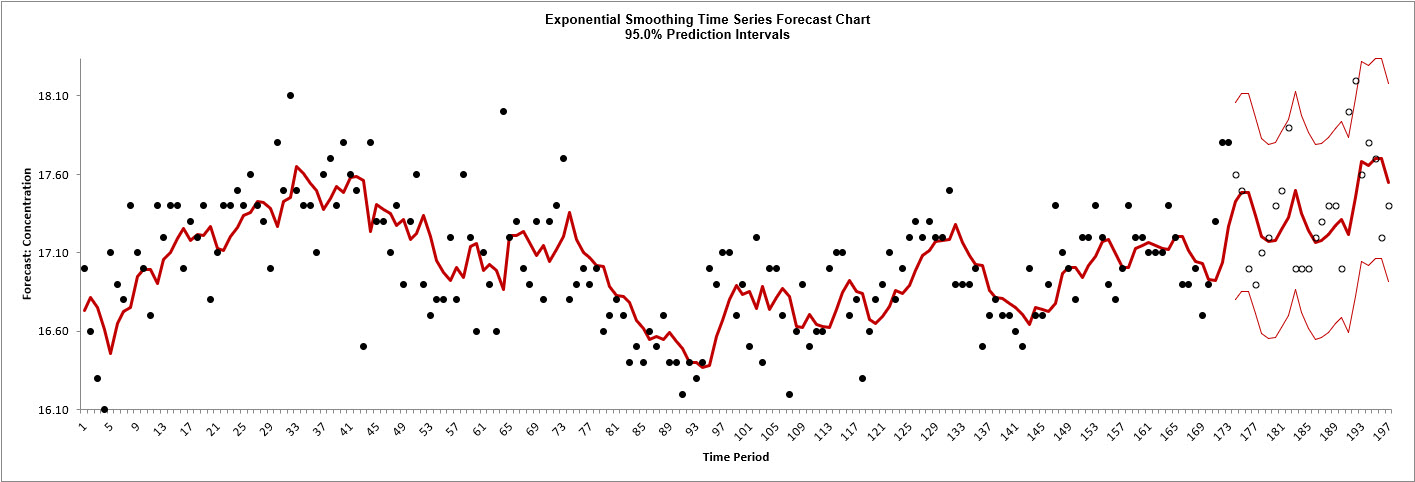The blank dots are the data values in the withhold sample with a one-step-ahead forecast and prediction intervals displayed at the start of the withhold sample.

41. Scroll down to view the Exponential Smoothing Model header: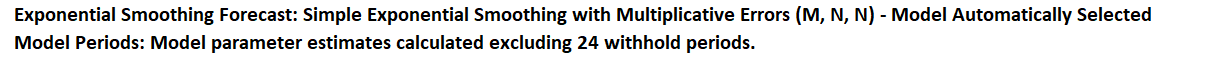As with the complete data, the model Simple Exponential Smoothing with Multiplicative Errors (M, N, N) was selected as the best fit for the Concentration data based on the AICc criterion. The header also includes the number of specified withhold periods.

42. The Parameter Estimates are: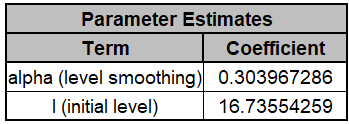These are close to the parameter estimates obtained above (which used all of the data with the multiplicative model).

43. The Exponential Smoothing Model Statistics are: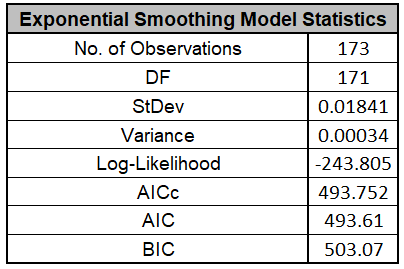These are fairly close to the model statistics obtained above (which used all of the data with the multiplicative model). Here we are using only 173 of the 197 observations.

44. The Forecast Accuracy metrics are:As expected, the Out-of-Sample (Withhold) One-Step-Ahead Forecast errors are larger than the In-Sample (Estimation) One-Step-Ahead Forecast errors.

45. The Forecast Table is given as:These are the same forecast and prediction interval values displayed in the Forecast Chart, but provided for further analysis or charting. The Withhold Data is also displayed.

46. The ACF/PACF/LB Residual Plots and Residual Plots are based on the in-sample data. The plots look similar to the complete data above, except for the Ljung-Box P-Values:The Simple Exponential Smoothing with Multiplicative Errors (M, N, N) model is a better fit to the subset than the complete data, with all P-Values being blue (> .05).The Simple Exponential Smoothing with Multiplicative Errors (M, N, N) model is a better fit to the subset than the complete data, with all P-Values being blue (> .05).

47. If Include in Residuals was checked then the residuals would also include the Out-of-Sample (Withhold) One-Step-Ahead Forecast errors.

48. Now we will rerun Exponential Smoothing on the Concentration data, but use Multi-Step-Ahead for the Withhold Forecast. Click Recall SigmaXL Dialog menu or press F3 to recall last dialog. Select Withhold Forecast Type: Multi-Step-Ahead with Prediction Interval at Start of Withhold.49. Click OK. The exponential smoothing forecast report is given: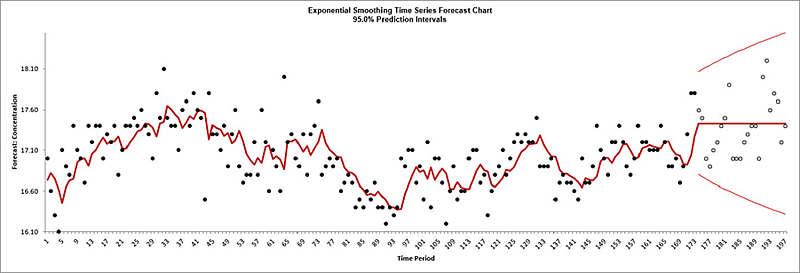The blank dots are the data values in the withhold sample with a multi-step forecast and prediction intervals displayed at the start of the withhold sample.

50. The Forecast Accuracy metrics are: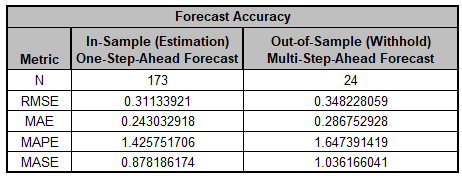As expected, the Out-of-Sample (Withhold) Multi-Step-Ahead Forecast errors are larger than the In-Sample (Estimation) One-Step-Ahead Forecast errors and the Out-of-Sample (Withhold) One-Step-Ahead Forecast errors above.

## Monthly Airline Passengers - Series G

1. Open Monthly Airline Passengers - Series G.xlsx (Sheet 1 tab). This is the Series G data from Box and Jenkins, monthly total international airline passengers for January 1949 to December 1960. See the Run Chart, ACF/PACF Plots, Spectral Density Plot and Seasonal Trend Decomposition Plots for this data.

2. Click SigmaXL > Time Series Forecasting > Exponential Smoothing Forecast > Forecast. Ensure that the entire data table is selected. If not, check Use Entire Data Table. Click Next.

3. Select Monthly Airline Passengers, click Numeric Time Series Data (Y) >>. Select Date, click Optional Time Axis Labels >>. Check Display ACF/PACF/LB Plots and Display Residual Plots. Check Specify Model Periods. Set Withhold Periods = 24 (i.e., we will forecast 24 months and compare to withheld actual). Select Withhold Forecast Type: Multi-Step-Ahead with Prediction Interval at Start of Withhold. Check Seasonal Frequency with Specify = 12. Check Box-Cox Transformation and select Rounded Lambda (selected because the Run Chart showed an increase in the seasonal variance over time). We will use the default Prediction Interval = 95.0%.• Withhold Forecast Type: Multi-Step-Ahead with Prediction Interval at Start of Withhold will exclude the withhold sample from automatic model identification and parameter estimation and does not use the withhold data to update the predicted one-step ahead forecast. This is useful to assess forecast error when you are interested in a long-term forecast window (horizon). The prediction interval will be displayed for the duration of the withhold sample. Note that the length of the prediction interval is determined by the number of withhold periods, so overrides the specified No. of Forecast Periods.

• Seasonal Frequency Specify is used to specify the seasonal frequency. Note that Exponential Smoothing is limited to a maximum seasonal frequency of 24. For higher frequencies use Exponential Smoothing – Multiple Seasonal Decomposition (MSD)

• Seasonal Frequency Select gives a drop-down list of commonly used seasonal frequencies:

•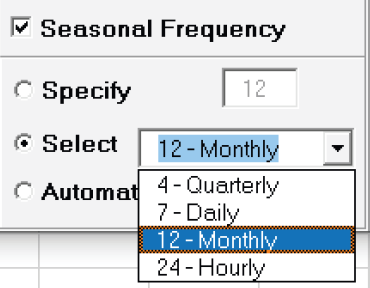• Seasonal Frequency Automatically Detect should be used if uncertain what the seasonal frequency value is (or do a Spectral Density Plot prior to the Seasonal Trend Decomposition Plots). If detected frequency is > 24, 1 is returned. If that occurs, please use Exponential Smoothing – Multiple Seasonal Decomposition (MSD).

• Box-Cox Transformation with Rounded Lambda will select Lambda = 0 (Ln), 0.5 (SQRT) or 1 (Untransformed). Threshold (Shift) is computed automatically if the time series data includes 0 or negative values, otherwise it is 0.

• Box-Cox Transformation with Optimal Lambda uses the range of 0 to 1 for Lambda. Threshold is computed automatically if the time series data includes 0 or negative values.

• Box-Cox Transformation with Lambda & Threshold (Shift) if left blank, will compute optimal lambda and threshold. The user may also specify Lambda and Threshold. Lambda may be specified outside of the 0 to 1 range, but practically for time series analysis, should be limited to -1 to 2. Threshold is typically 0, but if the time series data includes 0 or negative values, a negative threshold value should be entered that is smaller than the minimum data value. This value will be subtracted from the data resulting in positive time series values.

4. Click Model Options.

5.6. We will use the default Automatic Model Selection with AICc as the Model Selection Criterion. Click OK to return to the Exponential Smoothing Forecast dialog. Click OK. The exponential smoothing forecast report is given:

7.The blank dots are the data values in the withhold sample with a multi-step forecast and prediction intervals displayed at the start of the withhold sample. Note that the data has been transformed using Box-Cox, but the inverse transformation is applied to produce this chart with the original units.

8. Scroll down to view the Exponential Smoothing Model header:

9.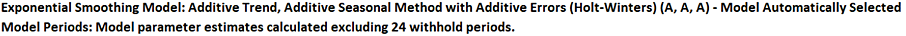The model Additive Trend, Additive Seasonal Method with Additive Errors (Holt-Winters) (A, A, A) was automatically selected as the best fit for the Box-Cox transformed Airline Passenger data based on the AICc criterion. Note that multiplicative models are not considered when Box-Cox Transformation is checked. If a multiplicative model was desired, then one could manually transform the data and model Ln(Airline Passengers) with Box-Cox Transformation unchecked.

The header also includes the number of specified withhold periods.

10. The Exponential Smoothing Model Information is given as:

11.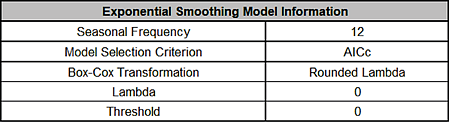12. The Parameter Estimates are:

13.• Error includes the smoothing parameter alpha and initial level value (l). The error is additive, but on the Ln transformed data.

• Trend adds a smoothing parameter (beta) and initial trend value (b).

• Seasonal adds a smoothing parameter (gamma) and initial seasonal values (s1 to s12), constrained to sum to zero for additive.

14. The Exponential Smoothing Model Statistics are:

15.• The number of observations, n = 144 – 24 (withhold) = 120

• Degrees of freedom (DF) = 120 (n) – 16 (terms in the model, excluding s12) = 104

• Note that the model statistics are based on the Ln transformed data, not the original data.

16. The Forecast Accuracy metrics are:

17.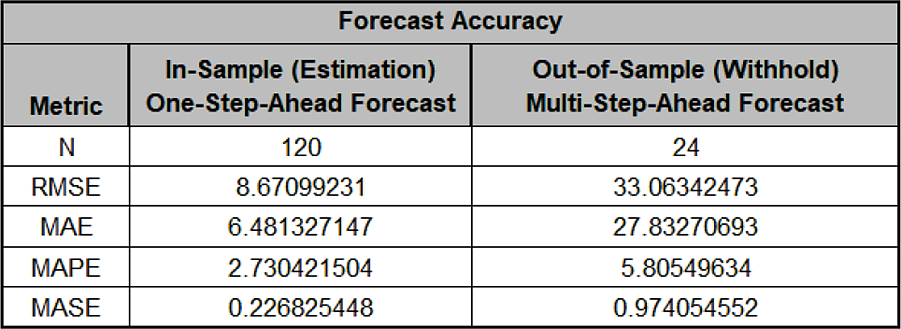As expected, the Out-of-Sample (Withhold) Multi-Step-Ahead Forecast errors are larger than the In-Sample (Estimation) One-Step-Ahead Forecast errors. Note, if we were primarily interested in a short term one-step ahead forecast, then we would have selected Withhold Forecast Type: One-Step-Ahead and this table would show Out-of-Sample (Withhold) One-Step-Ahead Forecast errors.

Forecast Accuracy metrics are calculated using the actual raw data versus inverse transformed forecast as displayed in the Forecast Chart and Table, so allows comparison across all model types and transformations.

18. Click on the Exp. Smooth. ACF PACF LB sheet to view the ACF/PACF/LB Plots:

19.The ACF/PACF Residuals Plots indicate that almost all of the autocorrelation has been accounted for in the model, but the Ljung-Box plot shows that significant autocorrelation still remains (the red P-Values are significant at alpha=.05) - so the model can potentially be improved. This does not mean that the model is a bad model, it can still be very useful for prediction purposes, but the prediction intervals may not provide accurate coverage.

20. Click on the Exp. Smoothing Residuals sheet to view the Residual Plots:

21.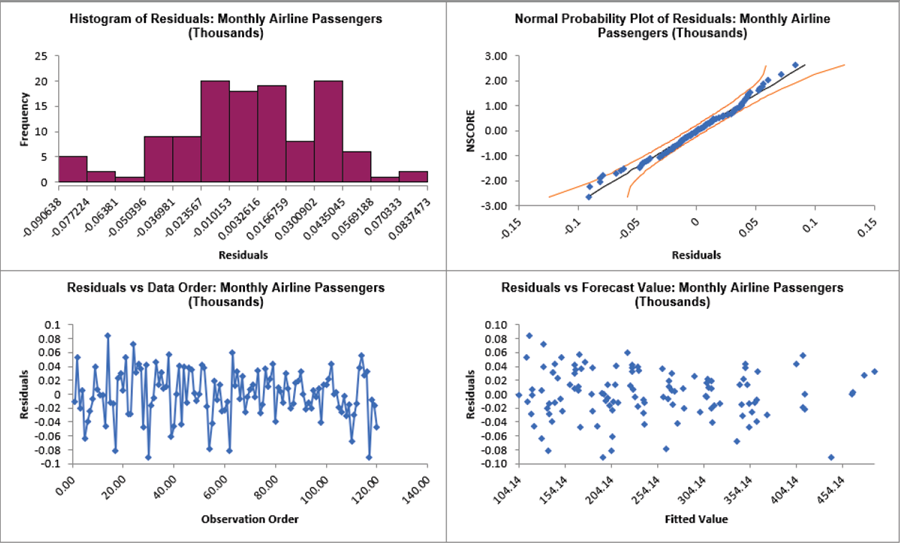The residuals are approximately normally distributed, with a roughly straight line on the normal probability plot. There are no obvious extreme outliers or patterns in the charts.

Note that the residuals are based on the Ln transformed data, not the original data. The Exponential Smoothing Model Information to the right of the plots shows the Box-Cox Transformation information.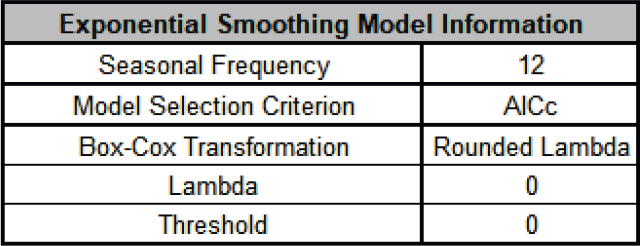# Web Demos

Our CTO and Co-Founder, John Noguera, regularly hosts free Web Demos featuring SigmaXL and DiscoverSim